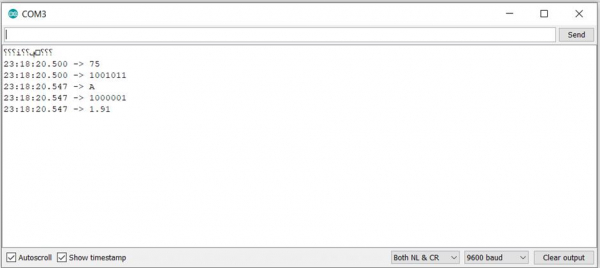# Print binary values in Arduino

In order to print binary representation of numbers/ characters in Arduino, you can add 'BIN' as the second argument of your Serial.print() function. Example is shown below −

## Example

void setup() {
// put your setup code here, to run once:
Serial.begin(9600);
Serial.println();
Serial.println(75);
Serial.println(75, BIN);
Serial.println('A');
Serial.println('A',BIN);
Serial.println(1.912,BIN);
}
void loop() {
// put your main code here, to run repeatedly:

}

The Serial Monitor output for the above code can be seen below −As you can see, this works only for integers and characters, and not for floating-point numbers. For characters, the binary value of the ASCII equivalent of that character is printed. You can access the ASCII table from here: https://www.cs.cmu.edu/~pattis/15-1XX/common/handouts/ascii.html. For negative integers, the results are a bit strange. For instance, Serial.print(-75,BIN) will output 11111111111111111111111110110101, which translates to a decimal value of 4294967221, which corresponds to (2^32 – 75). So essentially, the value printed corresponds to an integer overflow. Of course, this output will be seen on a board which uses 4 bytes (32 bits) to represent an integer. If you are using a board which used two bytes (16 bits) to represent an integer, the output will be (2^16 – 75).